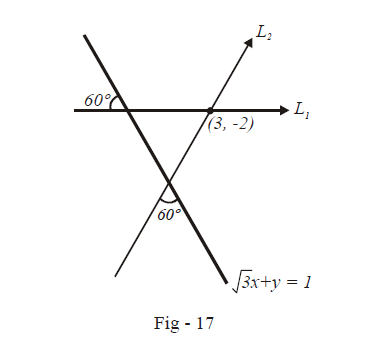# Examples on Point and Angle of Intersection of Two Straight Lines

Go back to  'Straight Lines'

Example – 5

Find the equation to the straight line which passes through (3, –2) and is inclined at $${{60}^{\circ }}$$ to the line $$\sqrt 3 \,x + y = 1.$$

Solution: Observe carefully that there will be two such lines. Denote the two lines by $${L_1}\,\,{\text{and}}\,\,{L_2}$$Let the slope of the line(s) we require be m.

The slope of $$\sqrt 3 \,x + y = 1$$ is $${m_1} = - \sqrt 3$$

Since we want the acute angle between the two lines to be 60º, we must have by Art - 5,

\begin{align} & \qquad\quad\tan 60{}^\text{o}=\left| \frac{{{m}_{1}}-m}{1+m{{m}_{1}}} \right| \\ & \Rightarrow \quad \sqrt{3}=\left| \frac{-\sqrt{3}-m}{1-\sqrt{3}\,m} \right| \\ & \Rightarrow \quad \frac{m+\sqrt{3}}{1-\sqrt{3}\,m}=\pm \,\sqrt{3} \\ & \Rightarrow\quad m+\sqrt{3}=\sqrt{3}-3m\,\,\text{or}\,\,m+\sqrt{3}=3m-\sqrt{3} \\ & \Rightarrow\quad m=0\,\,\text{or}\,\,\,m=\sqrt{3} \\ \end{align}

Since we get two values of m, this confirms our earlier assertion that two such lines will exist. We now have the slope. We also know that the lines pass through (3, –2). We can therefore use the point-slope form to write down the required equations:

\begin{align}& \qquad \qquad {{L}_{1}}\equiv y-\left( -2 \right)=0\left( x-3 \right);\,\,\,{{L}_{2}}\equiv y-\left( -2 \right)=\sqrt{3}\left( x-3 \right) \\ & \Rightarrow \qquad \,\,\,{{L}_{1}}\equiv y+2=0\,\,\,\,\text{and}\,\,\,{{L}_{2}}\equiv y-\sqrt{3}\,x\,\,+2+3\sqrt{3}=0 \end{align}

Example – 6

Find the equation of the straight line which passes through the point $$\left( a{{\cos }^{3}}\theta ,\,\,a{{\sin }^{3}}\theta \right)$$ and is perpendicular to the straight line $$x\sec \theta +y\,\text{cosec}\theta =a$$ .

Solution: The slope of the given line is \begin{align}{{m}_{1}}=-\frac{\sec \theta }{\text{cosec}\theta }=-\tan \theta \end{align}

Therefore, the slope of the line we require will be given by mwhere

\begin{align} & \qquad \,\,\,{{m}_{2}}=-\frac{1}{{{m}_{1}}} \\ & \Rightarrow \qquad {{m}_{2}}=\cot \theta \end{align}

We now know the slope of the line and we are also given a fixed point through which the line passes. We can therefore use the point-slope form to determine its equation:

\begin{align} & \qquad\qquad\quad\;\; y-a{{\sin }^{3}}\theta =\cot \theta \left( x-a{{\cos }^{3}}\theta \right) \\ & \Rightarrow \qquad x\cos \theta -y\sin \theta =a\left( -{{\sin }^{4}}\theta +{{\cos }^{4}}\theta \right) \\ & \qquad \qquad \qquad \qquad \qquad=a\left( {{\cos }^{2}}\theta +{{\sin }^{2}}\theta \right)\left( {{\cos }^{2}}\theta -{{\sin }^{2}}\theta \right) \\ & \qquad \qquad \qquad \qquad \qquad=a\cos 2\theta \end{align}

Thus, the required equation is

$x\cos \theta -y\sin \theta =a\cos 2\theta$